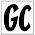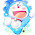### Challenge Problem: Taking a Quiz

I thought of this problem about two years ago and was going to send it out as the one of the weekly challenge problems for the ACM student chapter at UD, however I was unable to solve it when I first thought of it, and so I put it on the back-burner for a while.  Recently, I revisited it and made some progress on it, but was still unable to find a strategy that can solve it at all, let alone efficiently.  I am posting the problem to this blog in the hopes that you can help me figure it out by posting your ideas and code in the comments below.  Anyway, here is the problem:
You are taking a quiz with $n$ true/false questions that you didn't study for, but you are allowed to retake the quiz as many times as you want. Every time you take the quiz, you are told how many questions you got correct, but not which questions were right and wrong.  Your final score is the number of answers you got correct on your last submission minus the number of times you retook the quiz.  Create a program that will maximize your expected score on this quiz.
I'm going to quickly clarify some possible ambiguities:
• The quiz doesn't change between submissions.
• Every question is equally likely to be true/false.
• You believe all $2^n$ possible solutions are equally likely (because you didn't study).
• You cannot score below 0.
The first strategy that came to mind for me is very simple.  First, randomly (or deterministically, it doesn't really matter) fill in the answers and submit it for grading.  If you get at least 50% correct, accept that score.  Otherwise, swap all trues with falses and vice versa.  This strategy will guarantee that you will score at least a 50%, and it's a pretty easy counting problem to determine your exact expected score .  It turns our that your expected score converges to 50% as the number of questions grows to infinity though.

I'm confident there are many better strategies out there, and have thought of a couple myself, although I want to see what you can come up with.  I'd be very impressed if you can come up with a strategy that solves it exactly (guaranteed optimality) and in reasonable amount of time even for large $n$.  I'd also be interested in seeing other strategies you guys come up with, even if they aren't provably optimal.

To make it easy for you to create and test strategies, I coded up a small python script that simulates any test taking strategy for 10,000 20-question quizzes.  While it's fairly easy to exactly compute the expected score for a specific strategy (especially for the simple one I defined earlier), it's significantly harder to compute the expected score exactly for an arbitrary strategy.  That is why I am relying on simulations to approximate this expected value.  After 10,000 trials, the average should be close enough to the true expected value that we can trust it.

import numpy as np

#############################
## Do not modify this code ##
#############################

class Quiz:
def __init__(self, questions):
self.questions = questions
self.turns = 0
self.correct = 0
self.score = 0

def guess(self, guesses):
self.score = max(0, self.correct - self.turns)
self.turns += 1
return self.correct, self.score

def evaluate(quiz, quiz_taker):
quiz_taker(quiz.questions, quiz.guess)
return quiz.score

def simulate(quiz_taker, questions, trials):
return np.mean([evaluate(Quiz(questions), quiz_taker) for _ in range(trials)])

###########################################
## Write your quiz-taking functions here ##
###########################################

## Add more functions like this
guess = np.random.rand(questions) < 0.5 # guess the answers
# do nothing else - accept your current score

# Make random guess
# If at least 50% are correct, accept the score.
# Otherwise, invert every answer and guess again.
guess = np.random.rand(questions) < 0.5
if score*2 < questions:

students = [stub_student, two_guess]

for student in students:
print('%s: %0.4f' % (student.__name__, simulate(student, 20, 10000)))

Another similar question that I'd be interested in knowing the answer to is this:
Let $f_s(n)$ be the expected score for a quiz with $n$ questions under strategy $s$.  Does there exist a strategy $s$ such that $\lim_{n \rightarrow \infty} \frac{f_s(n)}{n} > \frac{1}{2}$?  If so, prove it.
If you come up with any good strategies, post them in the comments below!

1.As for when n is infinite, I think that is just similar as your scenario.

you just tried 20 "infinite times". You can think of n=infinite in groups of 20

-- guess = np.random.rand(questions) < 0.5
--prev_score = score
--index = 0
--step = 50
--while (index < questions):
----guess[index:(index+step)] = np.invert(guess[index:(index+step)])
----if(score +1 < prev_score ):
--------guess[index:(index+step)] = np.invert(guess[index:(index+step)])
----prev_score = score
----index = index + step

Hope is correct. But I'm not confident with my python.

The step 50 is between the divisors the one I found that maximizes the result.

So I really think it depends of the number of questions and how much are you taken in order to repeat the exam.

Kind regards.

1.Great idea! So I guess that settles the second question. I'm curious why 50 produces the best score - do you have any intuition behind why that is? Also, now that we've settled that question, my next question is what is the strategy that maximizes the value of the limit, and what is the limiting value? It's got to be something between 1/2 and 1 now.

2.This comment has been removed by the author.

3.Sorry I got some troubles with my previous post. I'm still don't know why I got better results with 50 in my previous post, but I'm affraid I was doing something wrong.

I made this little code to try to explain what I meant when I said 'So I really think it depends of the number of questions and how much are you taken in order to repeat the exam'

%matplotlib inline
import matplotlib
import numpy as np
import matplotlib.pyplot as plt
times = 1000
m = 150
penalty = 1
means = np.zeros(m)
for i in range(2,m):
--avg = np.zeros(times)
--for a in range(0,times):
----guess = np.random.rand(i)<0.5
----avg[a] = (np.abs(sum(guess)-(i-sum(guess))))/2.0
--means[i] = np.average(avg)
plt.plot(np.arange(1,m),(((np.arange(1,m))/2.0) + means[1:] - penalty)/np.arange(1,m))

4.Okay I think I see what you're saying about the penalty. For a penalty of 1, based on my interpretation of that plot, the optimal group size to use would be much less than 50. I actually analyzed the question theoretically and found the same thing. So that's very strange behavior. But your original code looks fine to me, and the quiz-taking code looks good to me as well, so I'm not sure how to explain this.

2.[url=http://rithven.pl/]REP[/url]
[url=http://rithven.pl/]blog podróżniczy[/url]
[url=http://rithven.pl/]ciekawe miejsca w Polsce[/url]
[url=http://rithven.pl/]gdzie na weekend[/url]
[url=http://rithven.pl/]zabytki w Polsce[/url]
[url=http://rithven.pl/]piękne miejsca w Polsce[/url]

3.4.